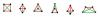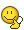BrainDen.com - Brain Teasers

## Question

Here's a quickie ... should take about a minute.

If you draw four points on a paper, no three of them collinear, they define six line segments.

If the points lie on the corners of a square, four of the segments are of length 1, say, and two are of length sqrt(2).

How many different configurations of four points give edges whose lengths are one of two values?

## Recommended Posts

• 0

a .

b c

. d

ac and bd are sqrt(2)

Edited by hhh3
##### Share on other sites

• 0

there are 4 such configurations: kite (2+4), equilateral triangle with the 4th point in the center (3+3), square (4+2), rhomboid made from 2 equilateral triangles (5+1)

##### Share on other sites

• 0

There are more.##### Share on other sites

• 0

Without any sort of formal proof, I think that these are all possible shapes. Although I haven't yet fully convinced myself that the last shape I drew actually exists. It seems like it should though.##### Share on other sites

• 0

Without any sort of formal proof, I think that these are all possible shapes. Although I haven't yet fully convinced myself that the last shape I drew actually exists. It seems like it should though.4points.jpg

Yes, these are the complete set.

The last one has a simple explanation.

Can you find it?

##### Share on other sites

• 0

The last one has a simple explanation.

Can you find it?

Do you know of any proof that this is a complete set?

I can prove it, although it's a bit brutish.

First, consider all cases in which no set of three points form an equilateral triangle.

Label the points A, B, C, and D, and name the distance from A to B as P. The other distance will be called Q. There must be at least one other line of length P or else there would be an equilateral triangle formed by BCD.

Consider the case where the other lines of length P do NOT involve points A or B. The only possible such scenario is if AB and CD are the only lines of length P. Then ABC and ABD must both be isosceles triangles with sides AC, BC, AD, and BD each of length Q. The only way to draw such a shape is to have C and D be on opposite sides of the line AB. Since length CD = AB = P, this forms the first shape I drew: a square with an X in the middle.

Now consider the case where at least one of the other lines of length P DOES involve points A or B. Define BC as another line with length P. If those were the only two lines of length P, then ACD would be an equilateral triangle with side length Q, so at least one of those three lines must be length P. AC cannot be length P or else ABC would be an equilateral triangle, so either line AD or CD must be length P. Hopefully it's clear that those are symmetric cases, so I'll say that line CD is length P. Note that lines AC and BD must be length Q in order to avoid forming equilateral triangles. So the only shape that fits these criteria has

AB P, BC P. CD P, AC Q, BD Q.

The only length not yet defined is legnth AD. If AD is length P, then you have the first shape I drew: the square with an X in the middle. If AD is length Q, then you have the last shape that I drew.

Now, consider all cases in which there is at least one set of three points that forms an equilateral triangle.

Define points A, B, and C as being part of such an equilateral triangle, and call the length of its sides P. Also note that point D must be either distance P or distance Q away from each of points A, B, and C, so there must be (at least) two points from which it is equidistant. Call those points A and B. We then know that D must lie somewhere along a line connecting C and the midpoint of AB.

Suppose that AD = BD = P. In that case, both ABC and ABD are equilateral with the same side lengths. It shouldn't take much proof to see that the second shape is the only possible one for this scenario.

So in all other cases, AD = BD must be length Q, and CD must be either P or Q. If CD is length Q, then it should also take very little proving to see that the third shape I drew is the only possible shape. If CD is length P, and we know that point D must lie along the line connecting C to the midpoint of AB, then there are only two possible positions for D: either distance P away from C going in the direction toward line AB - in which case you get the fifth figure I drew, or distance P away from C going in the direction away from line AB - in which case you get the fourth figure I drew.

Suppose my arms are short, and can just barely reach from one shoulder to the other. I start off with my arms crossed so each hand is touching the opposite shoulder. Then, keeping my arms within the plane formed by axes of my shoulders and my head, I rotate my arms upward at the same rate. The distance between my hands will gradually decrease from shoulder-width to zero, and the distance from my shoulder to its opposite hand will gradually increase from zero to shoulder-width. At some point, those two distances must become equal, and at that point my hand waving will have made the last figure.
##### Share on other sites

• 0

Without any sort of formal proof, I think that these are all possible shapes. Although I haven't yet fully convinced myself that the last shape I drew actually exists. It seems like it should though.4points.jpg

Yes, these are the complete set.

The last one has a simple explanation.

Can you find it?

A pentagon
##### Share on other sites

• 0

Ah, I see... a pentagon minus one point. Which I guess would be the only answer if there were five points instead of four.

Yes, I think it's the only five-point case.

When a friend asked me this question a few years ago, I was certain, after finding the first five, that I had them all.

Then he said, what if there were five points?I believe he said the problem came from a Jr. High. math competition that he had organized.

We ate the rest of our lunch in silence.

## Join the conversation

You can post now and register later. If you have an account, sign in now to post with your account.×   Pasted as rich text.   Paste as plain text instead

Only 75 emoji are allowed.

×   Your previous content has been restored.   Clear editor

×   You cannot paste images directly. Upload or insert images from URL.